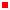Bionics Lab › Education > Classes

# Classes

 Instructor Jacob Rosen Office: Engineering IV Building, Room 37-146 Voice Office: 831.459.5302 e-mail: jacobrosen@ucla.eduMathematics of Engineering Course Summary: Methods of solving ordinary differential equations in engineering. Review of matrix algebra. Solutions of systems of first- and second-order ordinary differential equations. Introduction to Laplace transforms and their application to ordinary differential equations. Introduction to boundary value problems. Nonlinear differential equations and stability.Dynamics of Robotic Systems Course Summary: Motion planning and control of articulated dynamic systems: nonlinear joint control, experiments in joint control and multiaxis coordination, multibody dynamics, trajectory planning, motion optimization, dynamic performance and manipulator design, kinematic redundancies, motion planning of manipulators in space, obstacle avoidance. UCSC EE 154 CMPE 241 EE 241 CMPE 141Introduction to Feedback Control Systems Course Summary: Undergraduate-level (EE154) & graduate-level (CE 241) introduction to control of continuous linear systems using classical feedback techniques. Design of feedback controllers for command-following error, disturbance rejection, stability, and dynamic response specifications. Root locus and frequency response design techniques (Bode). Nyquist stability criterion. Design of dynamic compensators. Examples are drawn from electrical, mechanical, applications. Computer aided design with MATLAB. UCSC CMPE 9Introduction to Statics Dynamics & Biomechanics Course Summary: Theory and application of mathematical models to analyze statics and dynamics of mechanical and biomechanical systems (particles and rigid bodies) using a vector algebra. Covers: Newton's laws; free-body diagrams; structure analysis; friction; virtual work; energy and momentum methods; dynamics of bodies in two and three dimensions. UCSC CMPE 215Models of Robotic Manipulation Course Summary: Mathematical models of arbitrary articulated robotic as well as biological arms and their application to realistic arms and tasks, including the homogeneous coordinate model of positioning tasks, the forward and inverse kinematics models, the Jacobian Matrix, and the recursive Newton-Euler dynamic model.